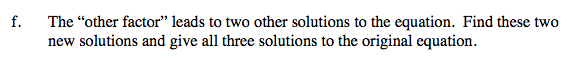### Home > A2C > Chapter 9 > Lesson 9.3.1 > Problem9-121

9-121.
1. Carlos is always playing games with his graphing calculator, but now his calculator has contracted a virus. The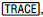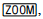and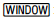functions on his calculator are not working. He needs to solve x3 + 5x2 − 16x −14 = 0, so he graphs y = x3 + 5x2 − 16x − 14 and sees the graph below in the standard window. Homework Help ✎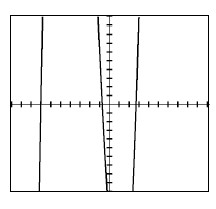1. From the graph, what appears to be an integer solution to the equation?

3. Since x = −7 is a solution to the equation, what is the factor associated with this solution?

4. Use polynomial division to find the other factor.

5. Use your new factor to complete this equation: Homework Help ✎
x3 + 5x2 − 16x −14 = (x + 7)(other factor) = 0

6. The “other factor” leads to two other solutions to the equation. Find these two new solutions and give all three solutions to the original equation.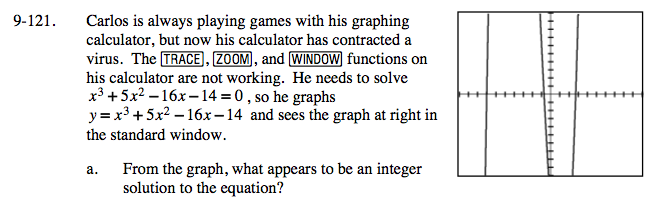x = −7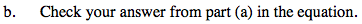y = −343 + 245 + 112 −14
y = 0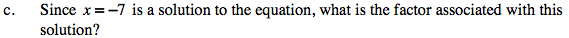(x + 7)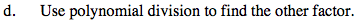Divide x3 + 5x2 −16x −14 by x + 7 using a generic rectangle.

x2 −2x −2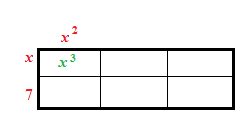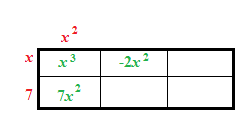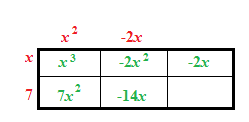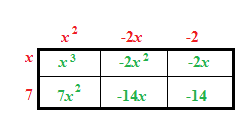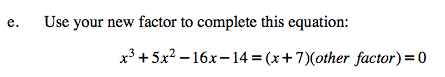x 3 + 5x 2 − 16x − 14 = (x + 7)(x 2 − 2x − 2) = 0